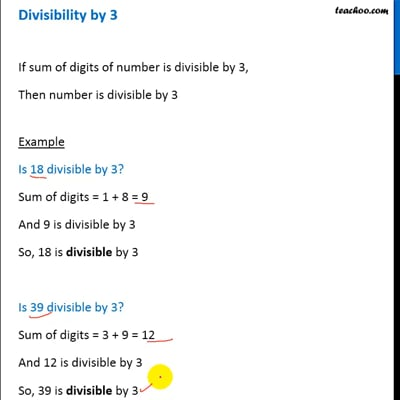Divisibility Tests - Divisibility by 3, 6, 9

Chapter 3 Class 6 Playing with Numbers
Concept wise

If sum of digits of number is divisible by 3,

Then number is divisible by 3

## Example

#### Is 18 divisible by 3?

Sum of digits = 1 + 8 = 9

And 9 is divisible by 3

So, 18 is divisible by 3

#### Is 39 divisible by 3?

Sum of digits = 3 + 9 = 12

And 12 is divisible by 3

So, 39 is divisible by 3

#### Is 93 divisible by 3?

Sum of digits = 9 + 3 = 12

And 12 is divisible by 3

So, 93 is divisible by 3

#### Is 270 divisible by 3?

Sum of digits = 2 + 7  + 0 = 9

And 9 is divisible by 3

So, 270 is divisible by 3

#### Is 25 divisible by 3?

Sum of digits = 2 + 5 = 7

But 7 is not divisible by 3

So, 25 is not divisible by 3

#### Is 37 divisible by 3?

Sum of digits  = 3 + 7 = 10

But 10 not divisible by 3

So, 37 is not divisible by 3

#### Is 260 divisible by 3?

Sum of digits = 2 + 6 + 0 = 8

But 8 is not divisible by 3

So, 260 is not divisible by 3This video is only available for Teachoo black users

Introducing your new favourite teacher - Teachoo Black, at only ₹83 per month